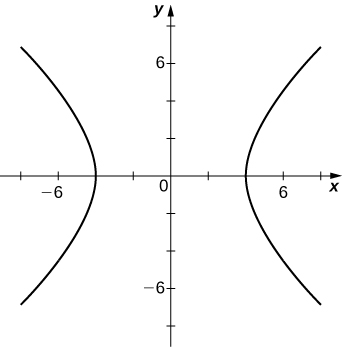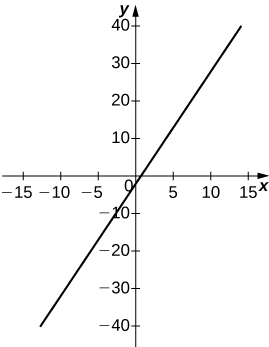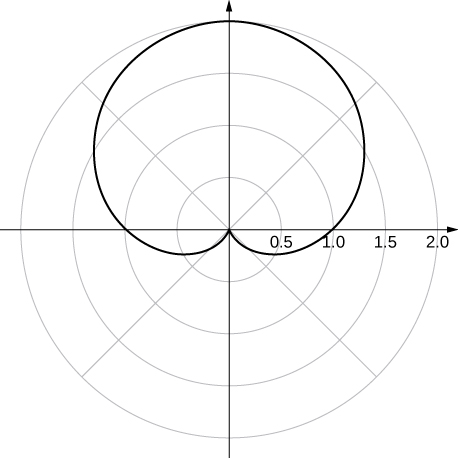$$\newcommand{\id}{\mathrm{id}}$$ $$\newcommand{\Span}{\mathrm{span}}$$ $$\newcommand{\kernel}{\mathrm{null}\,}$$ $$\newcommand{\range}{\mathrm{range}\,}$$ $$\newcommand{\RealPart}{\mathrm{Re}}$$ $$\newcommand{\ImaginaryPart}{\mathrm{Im}}$$ $$\newcommand{\Argument}{\mathrm{Arg}}$$ $$\newcommand{\norm}{\| #1 \|}$$ $$\newcommand{\inner}{\langle #1, #2 \rangle}$$ $$\newcommand{\Span}{\mathrm{span}}$$

# 4.3E:

$$\newcommand{\vecs}{\overset { \rightharpoonup} {\mathbf{#1}} }$$

$$\newcommand{\vecd}{\overset{-\!-\!\rightharpoonup}{\vphantom{a}\smash {#1}}}$$

## Polar Coordinates

In the following exercises, plot the point whose polar coordinates are given by first constructing the angle $$\displaystyle θ$$ and then marking off the distance r along the ray.

1) $$\displaystyle (3,\frac{π}{6})$$

Solution:2) $$\displaystyle (−2,\frac{5π}{3})$$

3) $$\displaystyle (0,\frac{7π}{6})$$

Solution:4) $$\displaystyle (−4,\frac{3π}{4})$$

5) $$\displaystyle (1,\frac{π}{4})$$

Solution:6) $$\displaystyle (2,\frac{5π}{6})$$

7) $$\displaystyle (1,\frac{π}{2})$$

Solution:For the following exercises, consider the polar graph below. Give two sets of polar coordinates for each point.8) Coordinates of point A.

9) Coordinates of point B.

Solution: $$\displaystyle B(3,\frac{−π}{3}) B(−3,\frac{2π}{3})$$

10) Coordinates of point C.

11) Coordinates of point D.

Solution: $$\displaystyle D(5,\frac{7π}{6}) D(−5,\frac{π}{6})$$

For the following exercises, the rectangular coordinates of a point are given. Find two sets of polar coordinates for the point in $$\displaystyle (0,2π]$$. Round to three decimal places.

12) $$\displaystyle (2,2)$$

13) $$\displaystyle (3,−4) (3, −4)$$

Solution: $$\displaystyle (5,−0.927)(−5,−0.927+π)$$

14) $$\displaystyle (8,15)$$

15) $$\displaystyle (−6,8)$$

Solution: $$\displaystyle (10,−0.927)(−10,−0.927+π)$$

16) $$\displaystyle (4,3)$$

17) $$\displaystyle (3,−\sqrt{3})$$

Solution: $$\displaystyle 2\sqrt{3},−0.524)(−2\sqrt{3},−0.524+π)$$

For the following exercises, find rectangular coordinates for the given point in polar coordinates.

18) $$\displaystyle (2,\frac{5π}{4})$$

19) $$\displaystyle (−2,\frac{π}{6})$$

Solution: $$\displaystyle −\sqrt{3},−1)$$

20) $$\displaystyle (5,\frac{π}{3})$$

21) $$\displaystyle (1,\frac{7π}{6})$$

Solution: $$\displaystyle (−\frac{\sqrt{3}}{2},\frac{−1}{2})$$

22) $$\displaystyle (−3,\frac{3π}{4})$$

23) $$\displaystyle (0,\frac{π}{2})$$

Solution: $$\displaystyle (0,0)$$

24) $$\displaystyle (−4.5,6.5)$$

For the following exercises, determine whether the graphs of the polar equation are symmetric with respect to the $$\displaystyle x$$-axis, the $$\displaystyle y$$ -axis, or the origin.

25) $$\displaystyle r=3sin(2θ)$$

Solution: Symmetry with respect to the x-axis, y-axis, and origin.

26) $$\displaystyle r^2=9cosθ$$

27) $$\displaystyle r=cos(\frac{θ}{5})$$

Solution: Symmetric with respect to x-axis only.

28) $$\displaystyle r=2secθ$$

29) $$\displaystyle r=1+cosθ$$

Solution: Symmetry with respect to x-axis only.

For the following exercises, describe the graph of each polar equation. Confirm each description by converting into a rectangular equation.

30) $$\displaystyle r=3$$

31) $$\displaystyle θ=\frac{π}{4}$$

Solution: Line $$\displaystyle y=x$$

32) $$\displaystyle r=secθ$$

33) $$\displaystyle r=cscθ$$

Solution: $$\displaystyle y=1$$

For the following exercises, convert the rectangular equation to polar form and sketch its graph.

34) $$\displaystyle x^2+y^2=16$$

35) $$\displaystyle x^2−y^2=16$$

Solution: Hyperbola; polar form $$\displaystyle r^2cos(2θ)=16$$ or $$\displaystyle r^2=16secθ.$$36) $$\displaystyle x=8$$

For the following exercises, convert the rectangular equation to polar form and sketch its graph.

37) $$\displaystyle 3x−y=2$$

Solution: $$\displaystyle r=\frac{2}{3cosθ−sinθ}$$38) $$\displaystyle y^2=4x$$

For the following exercises, convert the polar equation to rectangular form and sketch its graph.

39) $$\displaystyle r=4sinθ$$

40) $$\displaystyle x^2+y^2=4y$$

Solution:41) $$\displaystyle r=6cosθ$$

42) $$\displaystyle r=θ$$

Solution: $$\displaystyle xtan\sqrt{x^2+y^2}=y$$43) $$\displaystyle r=cotθcscθ$$

For the following exercises, sketch a graph of the polar equation and identify any symmetry.

44) $$\displaystyle r=1+sinθ$$

Solution: y-axis symmetry45) $$\displaystyle r=3−2cosθ$$

46) $$\displaystyle r=2−2sinθ$$

Solution: y-axis symmetry47) $$\displaystyle r=5−4sinθ$$

48) $$\displaystyle r=3cos(2θ)$$

Solution: x- and y-axis symmetry and symmetry about the pole49) $$\displaystyle r=3sin(2θ)$$

50) $$\displaystyle r=2cos(3θ)$$

Solution: x-axis symmetry51) $$\displaystyle r=3cos(\frac{θ}{2})$$

52) $$\displaystyle r^2=4cos(\frac{2}{θ})$$

Solution: x- and y-axis symmetry and symmetry about the pole53) $$\displaystyle r^2=4sinθ$$

54) $$\displaystyle r=2θ$$

Solution: no symmetry55) [T] The graph of $$\displaystyle r=2cos(2θ)sec(θ).$$ is called a strophoid. Use a graphing utility to sketch the graph, and, from the graph, determine the asymptote.

56) [T] Use a graphing utility and sketch the graph of $$\displaystyle r=\frac{6}{2sinθ−3cosθ}$$.

Solution: a line57) [T] Use a graphing utility to graph $$\displaystyle r=\frac{1}{1−cosθ}$$.

58) [T] Use technology to graph $$\displaystyle r=e^{sin(θ)}−2cos(4θ)$$.

Solution:59) [T] Use technology to plot $$\displaystyle r=sin(\frac{3θ}{7})$$ (use the interval $$\displaystyle 0≤θ≤14π$$).

60) Without using technology, sketch the polar curve $$\displaystyle θ=\frac{2π}{3}$$.

Solution:61) [T] Use a graphing utility to plot $$\displaystyle r=θsinθ$$ for $$\displaystyle −π≤θ≤π$$.

62) [T] Use technology to plot $$\displaystyle r=e^{−0.1θ}$$ for $$\displaystyle −10≤θ≤10.$$

Solution:63) [T] There is a curve known as the “Black Hole.” Use technology to plot $$\displaystyle r=e^{−0.01θ}$$ for $$\displaystyle −100≤θ≤100$$.

64) [T] Use the results of the preceding two problems to explore the graphs of $$\displaystyle r=e^{−0.001θ}$$ and $$\displaystyle r=e^{−0.0001θ}$$ for $$\displaystyle |θ|>100$$.

Solution: Answers vary. One possibility is the spiral lines become closer together and the total number of spirals increases.